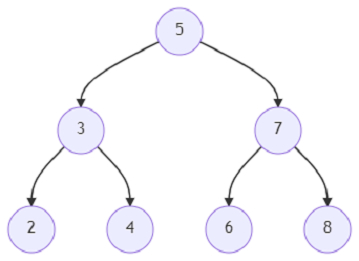# Program to find out the lowest common ancestor of a binary tree of given nodes using Python

Suppose, we are given a binary tree and are asked to find out the lowest common ancestor of all the nodes in the tree. The lowest common ancestor in a binary tree is the lowest node of which the nodes x1, x2, x3,...., xn are descendants. A particular node can also be a descendant of itself. We have to find the node and return it as an output. The inputs are the root node of the tree and the list of nodes that we have to find the ancestor of.

So, if the input is likeand the list of nodes that we have to find the ancestors of are [6, 8]; then the output will be 7.

The output is 7, because the lowest node of which the nodes 6 and 8 are descendants is 7.

To solve this, we will follow these steps −

• Define a function fn() . This will take node

• if node is similar to null, then

• return node

• otherwise when node is preset in nodes, then

• return node

• left := fn(left of node) ,

• right := fn(right of node)

• if left and right are not null, then

• return node

• otherwise,

• if left or right are not null, then

• return node

• nodes := a new list

• for each elem in node_list, do

• insert search_node(root, elem) at the end of nodes

• nodes := a new set from nodes

• return fn(root)

Let us see the following implementation to get better understanding −

## Example

import collections
class TreeNode:
def __init__(self, data, left = None, right = None):
self.data = data
self.left = left
self.right = right
def insert(temp,data):
que = []
que.append(temp)
while (len(que)):
temp = que
que.pop(0)
if (not temp.left):
if data is not None:
temp.left = TreeNode(data)
else:
temp.left = TreeNode(0)
break
else:
que.append(temp.left)
if (not temp.right):
if data is not None:
temp.right = TreeNode(data)
else:
temp.right = TreeNode(0)
break
else:
que.append(temp.right)
def make_tree(elements):
Tree = TreeNode(elements)
for element in elements[1:]:
insert(Tree, element)
return Tree
def search_node(root, element):
if (root == None):
return None
if (root.data == element):
return root
res1 = search_node(root.left, element)
if res1:
return res1
res2 = search_node(root.right, element)
return res2
def print_tree(root):
if root is not None:
print_tree(root.left)
print(root.data, end = ', ')
print_tree(root.right)
def solve(root, node_list):
nodes = []
for elem in node_list:
nodes.append(search_node(root, elem))
nodes = set(nodes)
def fn(node):
if not node:
return node
elif node in nodes:
return node
left, right = fn(node.left), fn(node.right)
return node if left and right else left or right
return fn(root)
root = make_tree([5, 3, 7, 2, 4, 6, 8])
print(solve(root, [6,8]).data)

## Input

make_tree([5, 3, 7, 2, 4, 6, 8]), [6, 8]

## Output

7